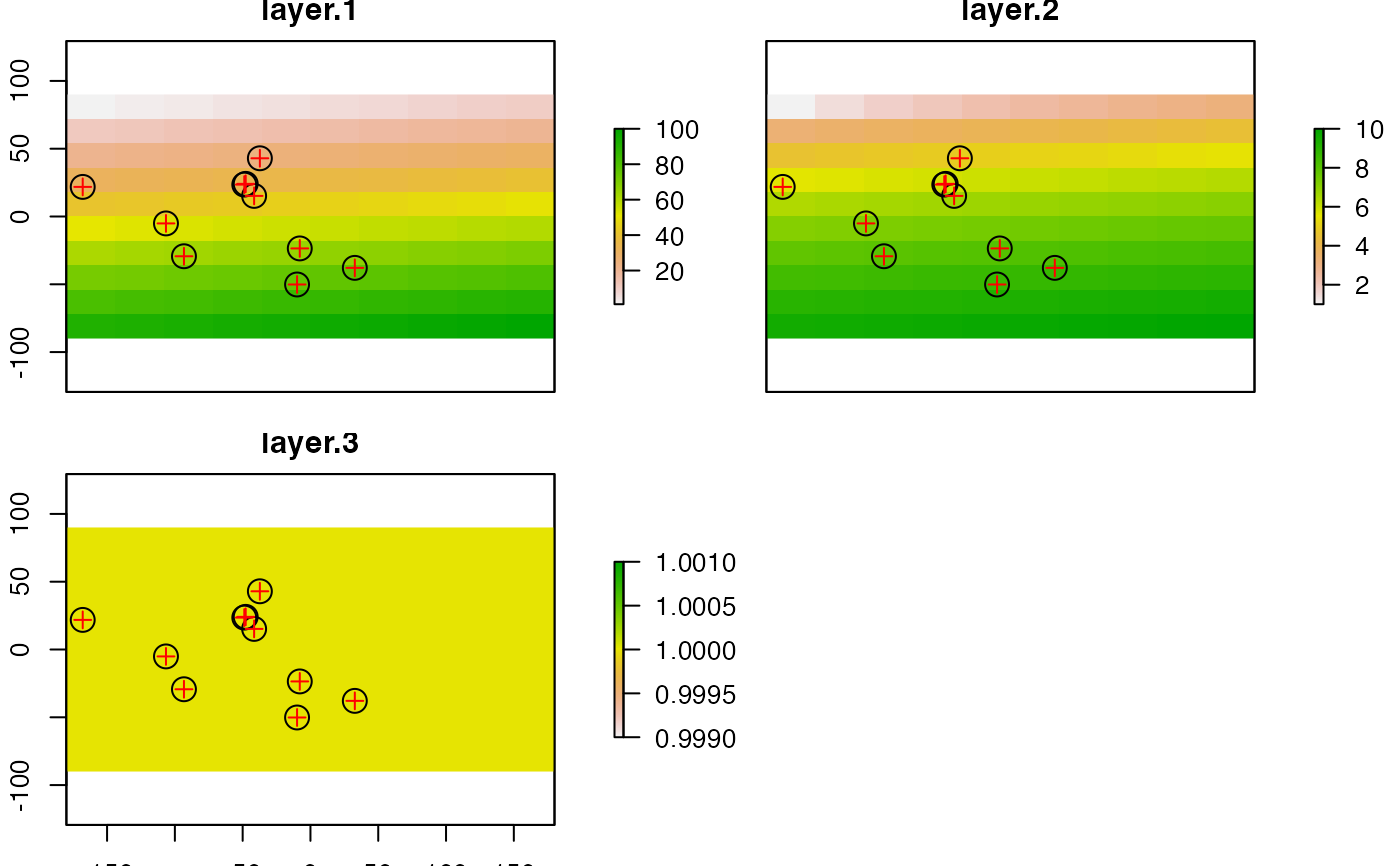Plot (that is, make a map of) the values of a Raster* object, or make a scatterplot of their values.

Points, lines, and polygons can be drawn on top of a map using plot(..., add=TRUE), or with functions like points, lines, polygons

See the rasterVis package for more advanced (trellis/lattice) plotting of Raster* objects.

# S4 method for Raster,ANY
plot(x, y, maxpixels=500000, col, alpha=NULL,
addfun=NULL, nc, nr, maxnl=16, main, npretty=0, ...)

# S4 method for Raster,Raster
plot(x, y, maxpixels=100000, cex, xlab, ylab, nc, nr,
maxnl=16, main, add=FALSE, gridded=FALSE, ncol=25, nrow=25, ...)

## Arguments

x

Raster* object

y

If x is a RasterStack or RasterBrick: integer, character (layer name(s)), or missing to select which layer(s) to plot. If missing, all RasterLayers in the RasterStack will be plotted (up to a maximum of 16). Or another Raster* object of the same extent and resolution, to produce a scatter plot of the cell values.

maxpixels

integer > 0. Maximum number of cells to use for the plot. If maxpixels < ncell(x), sampleRegular is used before plotting. If gridded=TRUE maxpixels may be ignored to get a larger sample

col

A color palette, i.e. a vector of n contiguous colors generated by functions like rainbow, heat.colors, topo.colors, bpy.colors or one or your own making, perhaps using colorRampPalette. If none is provided, rev(terrain.colors(255)) is used unless x has a 'color table'

alpha

Number between 0 and 1 to set transparency. 0 is entirely transparent, 1 is not transparent (NULL is equivalent to 1)

colNA

The color to use for the background (default is transparent)

ext

An extent object to zoom in a region (see also zoom and crop(x, drawExtent())

useRaster

If TRUE, the rasterImage function is used for plotting. Otherwise the image function is used. This can be useful if rasterImage does not work well on your system (see note)

interpolate

Logical. Should the image be interpolated (smoothed)? Only used when useRaster = TRUE

Function to add additional items such as points or polygons to the plot (map). Typically containing statements like "points(xy); plot(polygons, add=TRUE)". This is particularly useful to add something to each map when plotting a multi-layer Raster* object.

npretty

integer. Number of decimals for pretty lables on the axes

...

Graphical parameters. Any argument that can be passed to image.plot and to base plot, such as axes=FALSE, main='title', ylab='latitude'

xlab

Optional. x-axis label)

ylab

Optional. y-axis label)

nc

Optional. The number of columns to divide the plotting device in (when plotting multiple layers in a RasterLayer or RasterBrick object)

nr

Optional. The number of rows to divide the plotting device in (when plotting multiple layers in a RasterLayer or RasterBrick object)

maxnl

integer. Maximum number of layers to plot (for a multi-layer object)

main

character. Main plot title

cex

Symbol size for scatter plots

gridded

logical. If TRUE the scatterplot is gridded (counts by cells)

ncol

integer. Number of columns for gridding

nrow

integer. Number of rows for gridding

## Details

Most of the code for the plot function for a single Raster* object was taken from image.plot (fields package).

Raster objects with a color-table (e.g. a graphics file) are plotted according to that color table.

## Note

raster uses rasterImage from the graphics package. For unknown reasons this does not work on Windows Server and on a few versions of Windows XP. On that system you may need to use argument useRaster=FALSE to get a plot.

The rasterVis package has lattice based methods for plotting Raster* objects (like spplot)

red-green-blue plots (e.g. false color composites) can be made with plotRGB

barplot, hist, text, persp, contour, pairs

## Examples

# RasterLayer
r <- raster(nrows=10, ncols=10)
r <- setValues(r, 1:ncell(r))
plot(r)

e <- extent(r)
e <- e / 2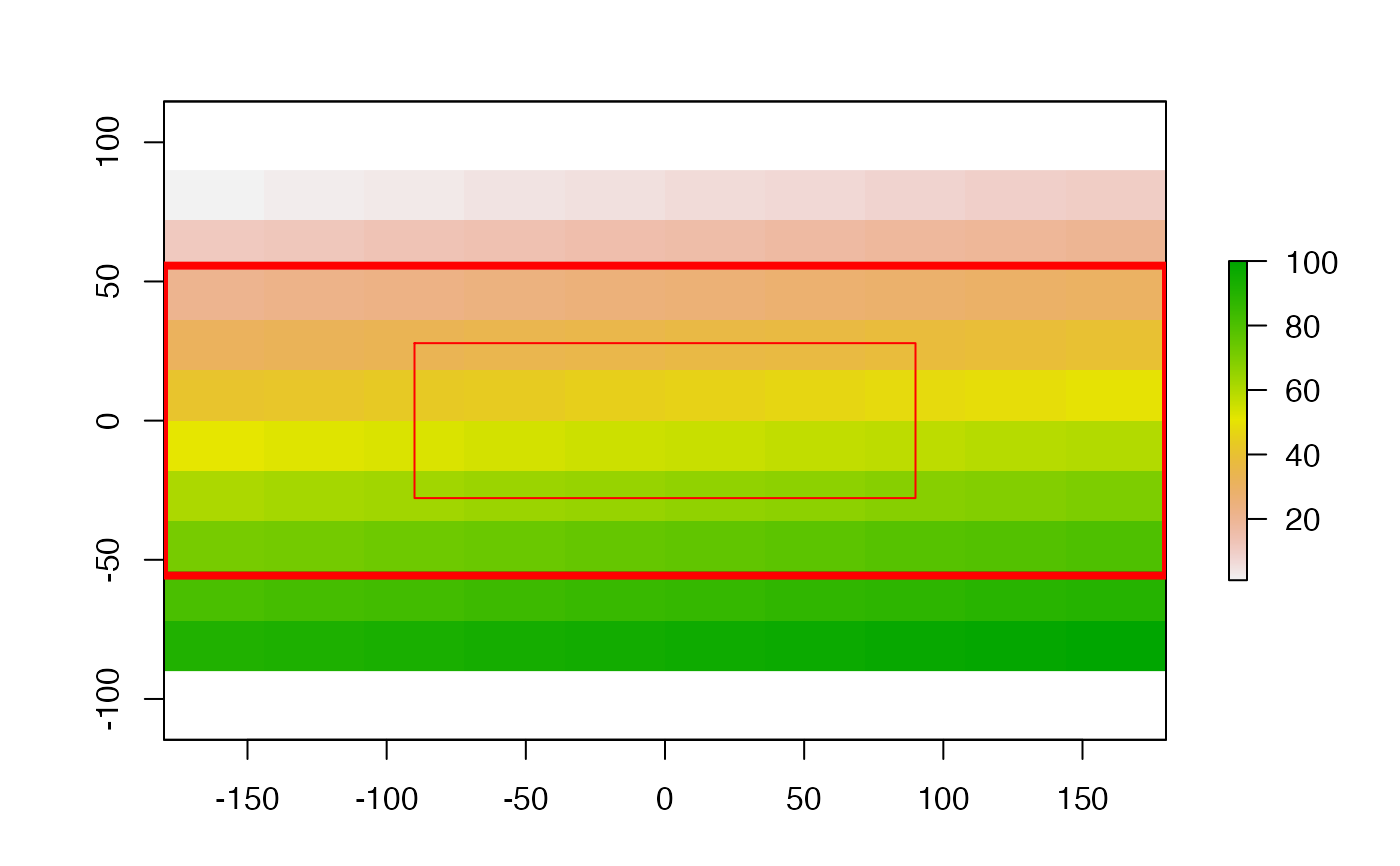# Scatterplot of 2 RasterLayers
r2 <- sqrt(r)
plot(r, r2)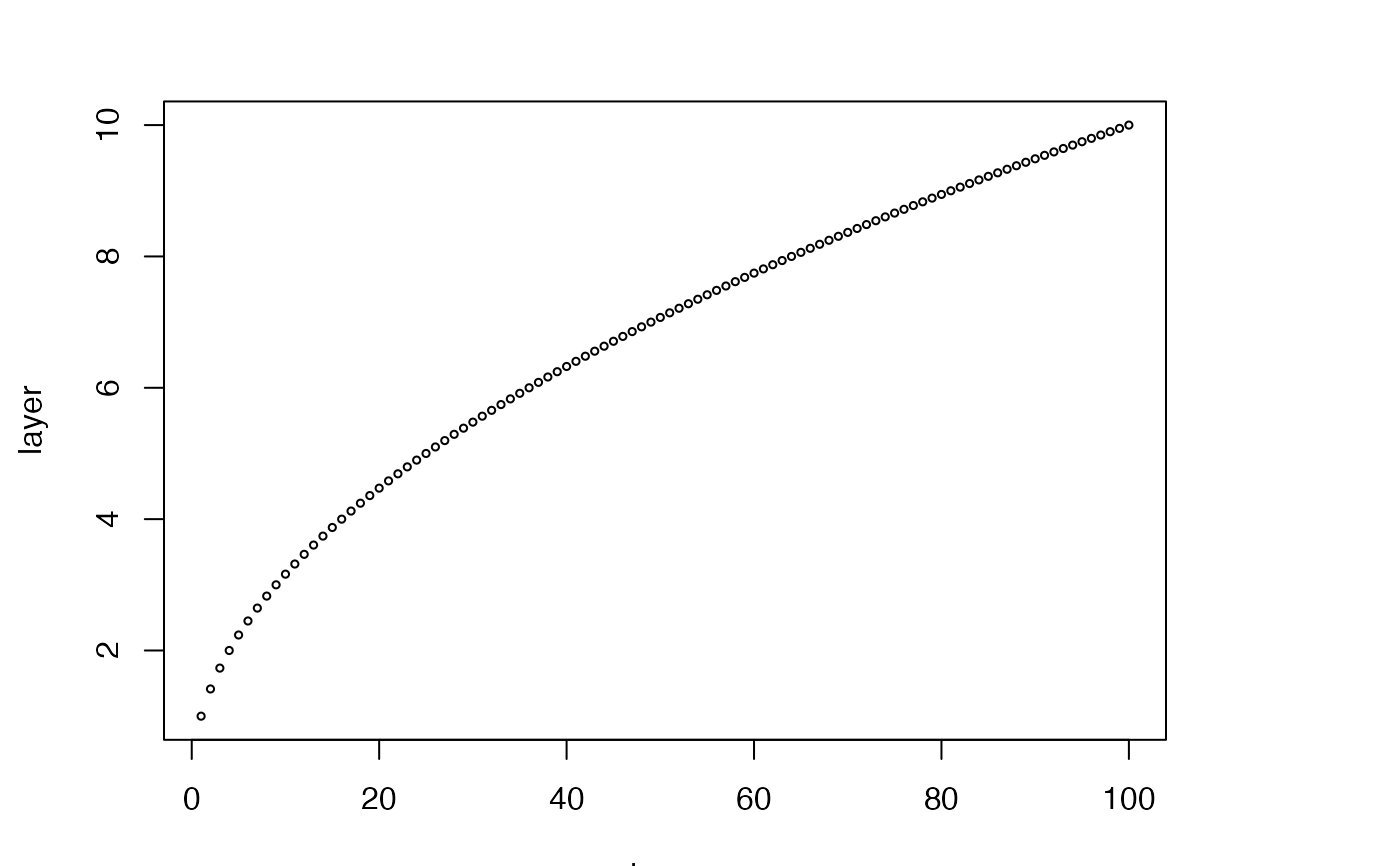plot(r, r2, gridded=TRUE)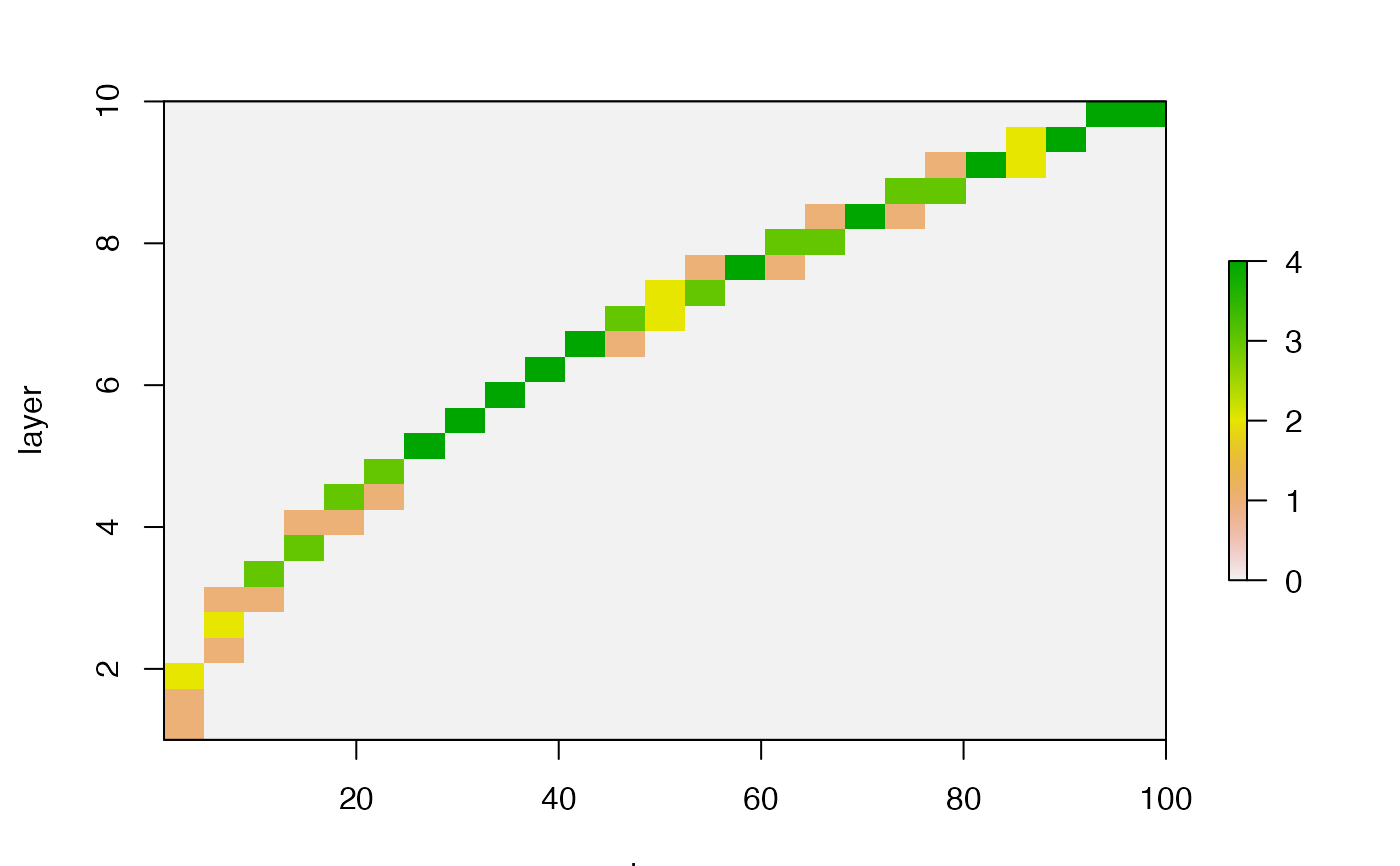# Multi-layer object (RasterStack / Brick)
s <- stack(r, r2, r/r)
plot(s, 2)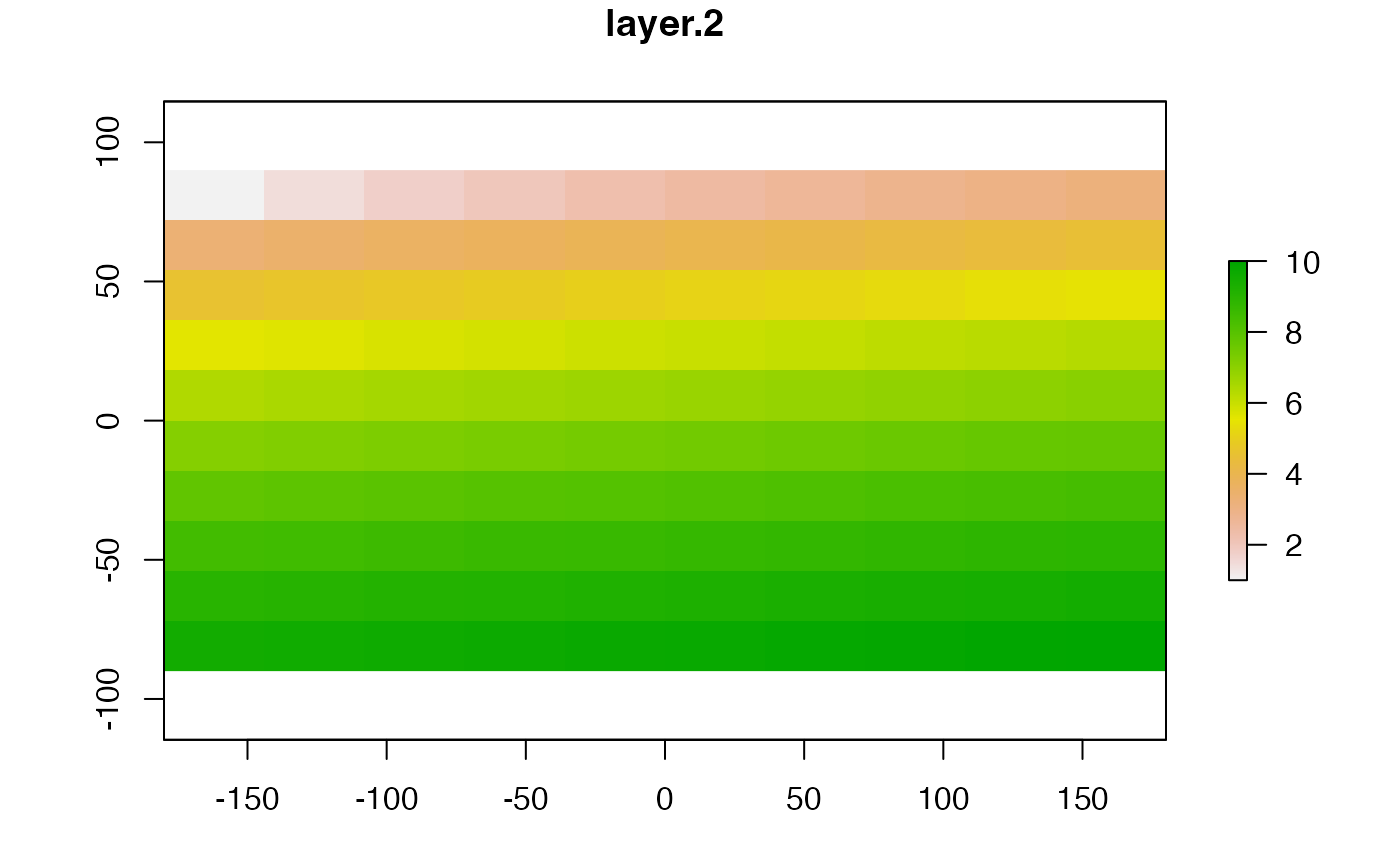plot(s)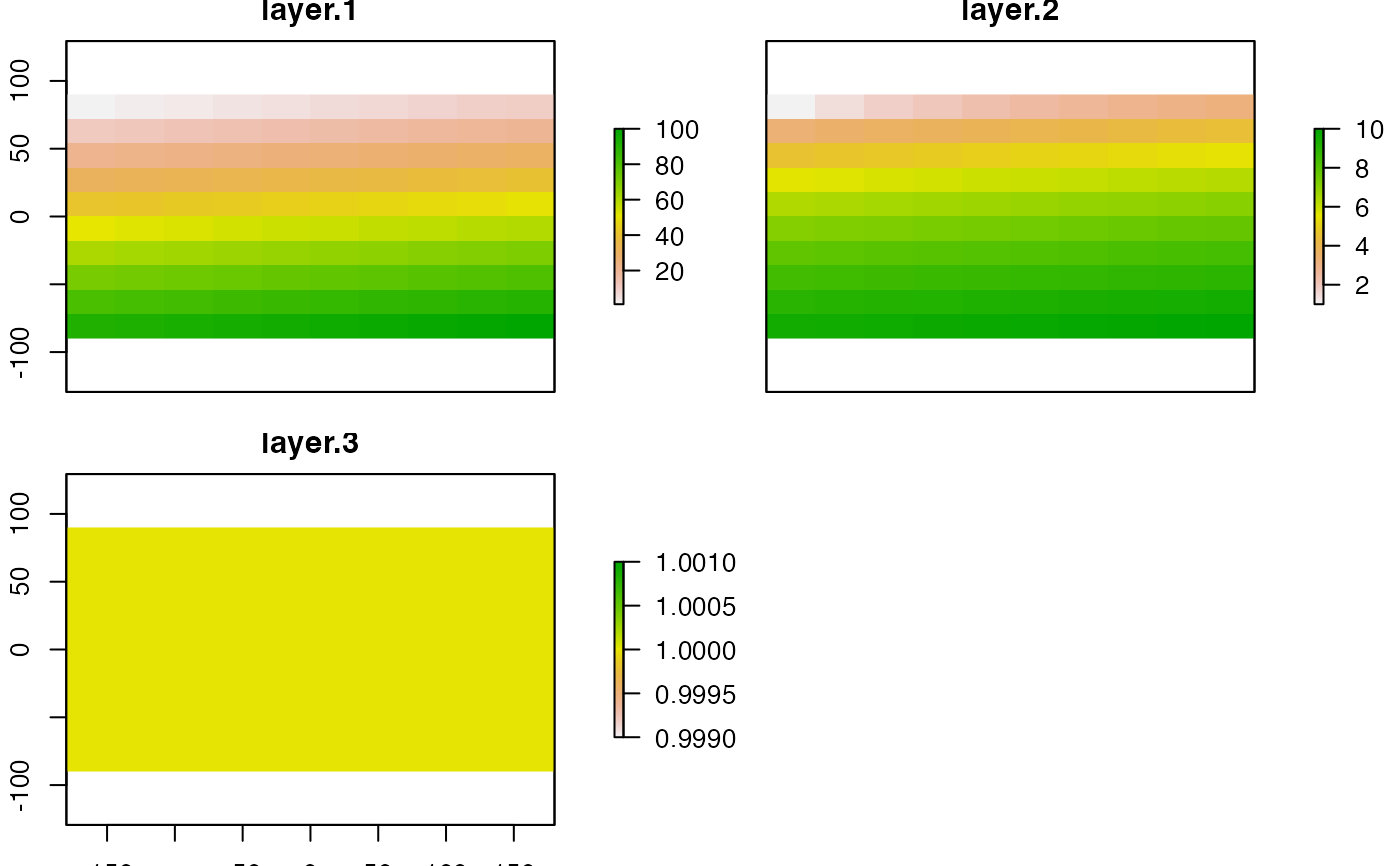# two objects, different range, one scale:
values(r) <- runif(ncell(r))
r2 <- r/2
brks <- seq(0, 1, by=0.1)
nb <- length(brks)-1
cols <- rev(terrain.colors(nb))
par(mfrow=c(1,2))
plot(r, breaks=brks, col=cols, lab.breaks=brks, zlim=c(0,1), main='first')
plot(r2, breaks=brks, col=cols, lab.breaks=brks, zlim=c(0,1), main='second')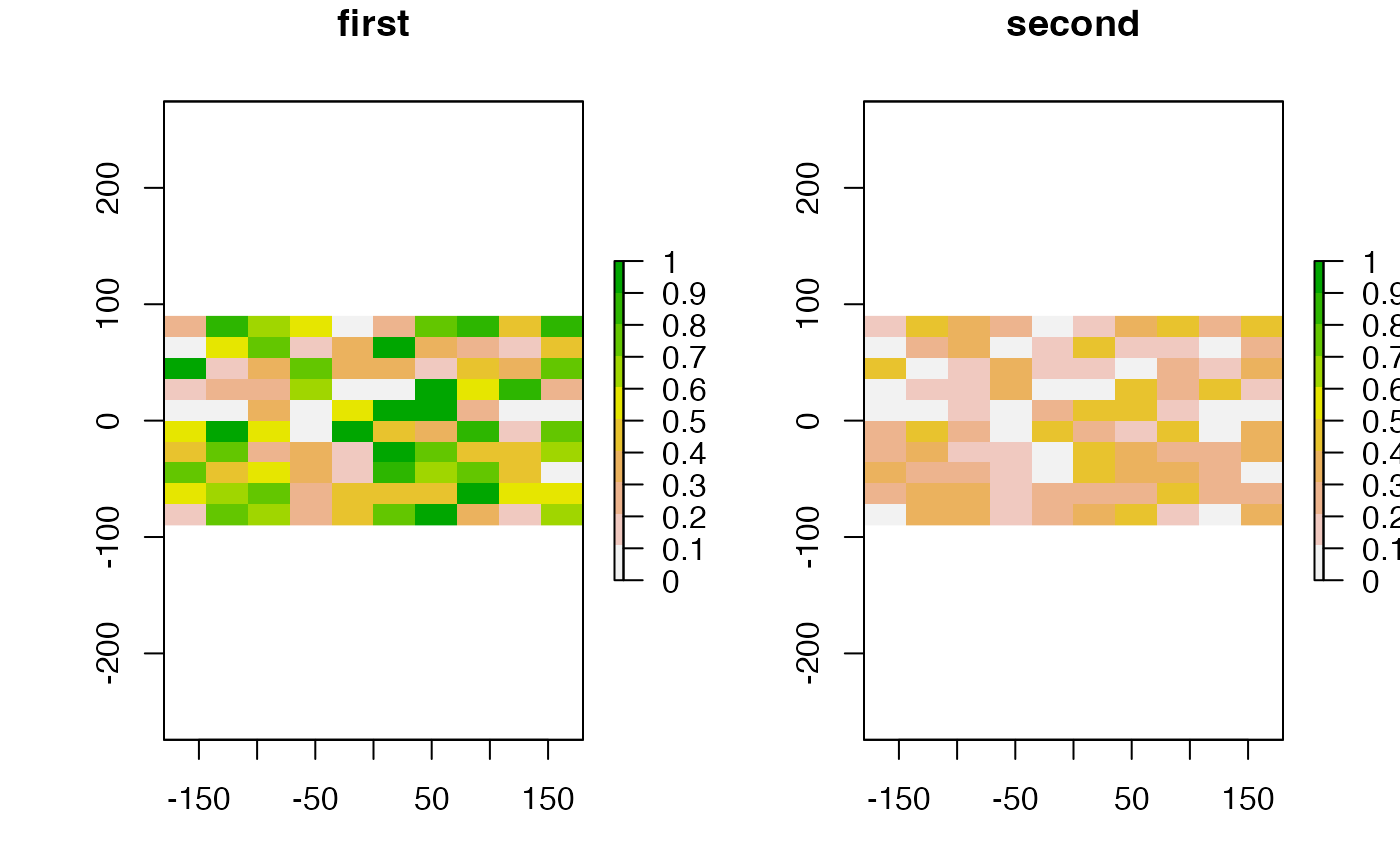# breaks and labels
x <- raster(nc=10, nr=10)
values(x) <- runif(ncell(x))
brk <- c(0, 0.25, 0.75, 1)
arg <- list(at=c(0.12,0.5,0.87), labels=c("Low","Med.","High"))
plot(x, col=terrain.colors(3), breaks=brk)
plot(x, col=terrain.colors(3), breaks=brk, axis.args=arg)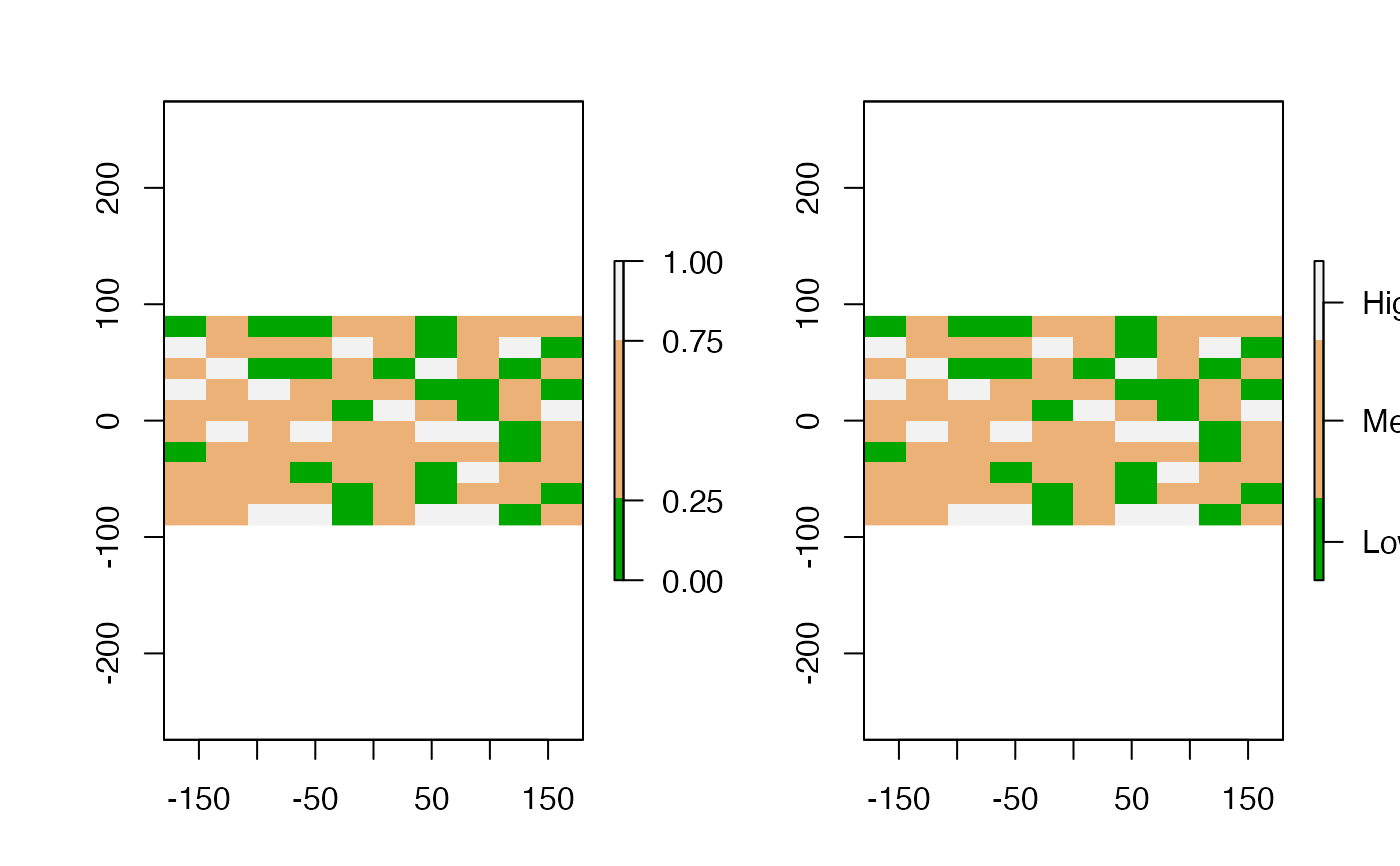par(mfrow=c(1,1))

# color ramp
plot(x, col=colorRampPalette(c("red", "white", "blue"))(255))

# adding random points to the map
xy <- cbind(-180 + runif(10) * 360, -90 + runif(10) * 180)
points(xy, pch=3, cex=5)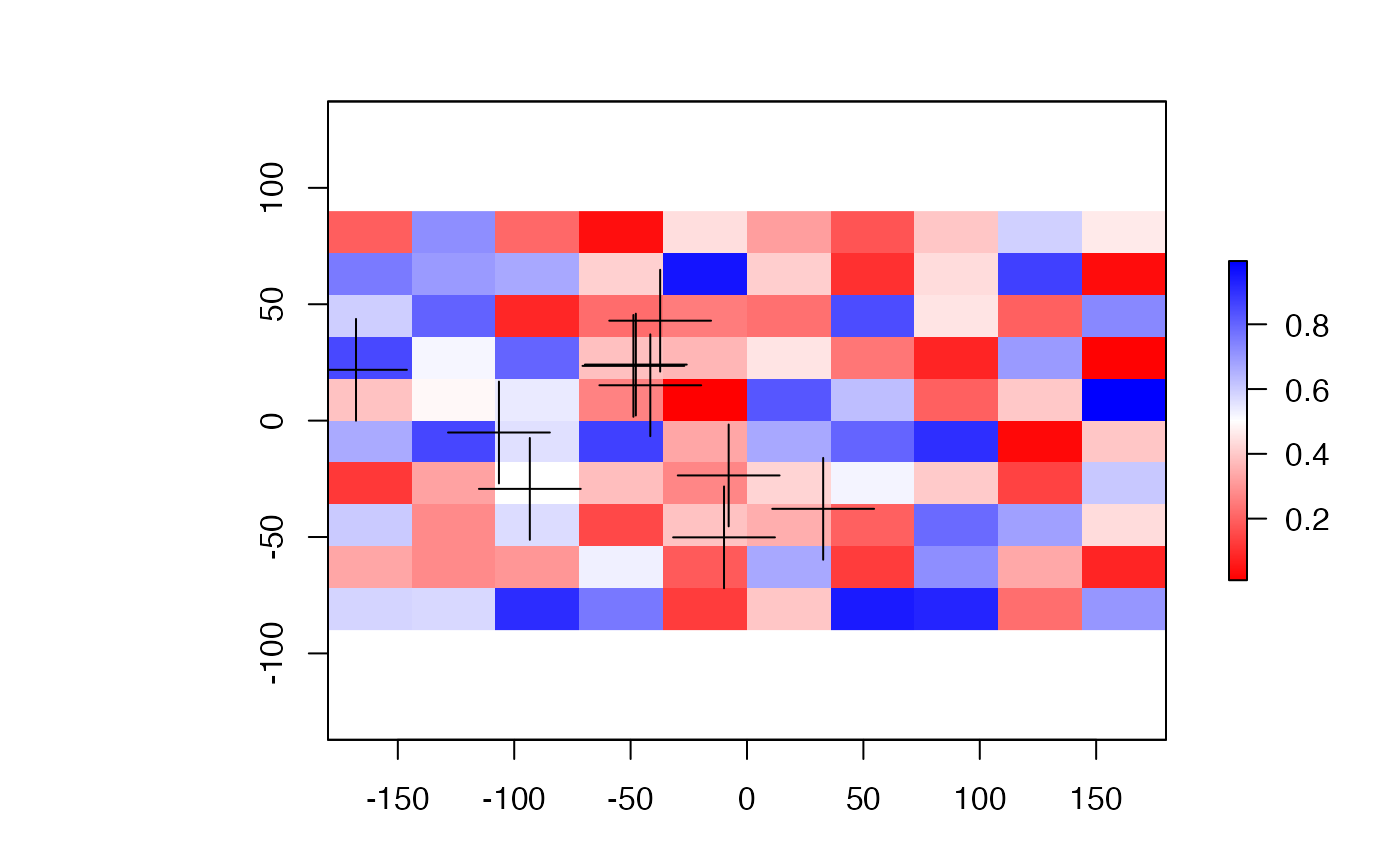# for SpatialPolygons do